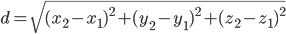# Generating an image with every RGB color value

At time I was writing code in processing to generate organic looking structures.```ArrayList myBugs = new ArrayList();
void setup()
{
size(500, 500);
frameRate(60);
background(0, 0, 0);
colorMode(HSB, 255);
color seedColor = color(200, 255, 255);
Bug seedBug = new Bug(int(width/2),int(height/2),seedColor);
}

void draw()
{
for(int i = 0; i<myBugs.size();i++)
{
myBugs.get(i).update();
}
if(frameCount%10==0)
{
saveFrame("Frame###.jpg");
}
}

class Bug
{
int xpos, ypos;
color myColor;
Bug(int x, int y, color c)
{
xpos = x;
ypos = y;
myColor = c;
}

void update()
{
int huemin = 0;
int huemax = 255;
int randChance = 45;
set(xpos, ypos, myColor);
if(get(xpos-1, ypos) == color(0, 0, 0))
{
if(random(100)<randChance)
{
myBugs.add(new Bug(xpos-1, ypos,color(random(huemin, huemax), 255, 255)));
}
}
if(get(xpos+1, ypos) == color(0, 0, 0))
{
if(random(100)<randChance)
{
myBugs.add(new Bug(xpos+1, ypos,color(random(huemin, huemax), 255, 255)));
}
}
if(get(xpos, ypos-1) == color(0, 0, 0))
{
if(random(100)<randChance)
{
myBugs.add(new Bug(xpos, ypos-1,color(random(huemin, huemax), 255, 255)));
}
}
if(get(xpos, ypos+1) == color(0, 0, 0))
{
if(random(100)<randChance)
{
myBugs.add(new Bug(xpos, ypos+1,color(random(huemin, huemax), 255, 255)));
}
}
println(myBugs.size());
if(myBugs.size()>10)
{
myBugs.remove(this);
return;
}
return;
}
}
```

It starts out by generating a seed “cell” the cell then randomly generates neighboring cells. The seed cell then deletes it self. All the newly generated cells then pick a random color, and repeat the same processes as taken by the seed cell. Later I decided to base the color on the number of pixels colored in. It does not use every color once, but it does go through all the possible hues.

I can vary the general shape by changing the percent chance for a cell to create a neighbor.Now for the fun part, getting every color to show up once, and only once. Computers represent colors as three distinct values red, green, and blue. Typically these values have a range of 0 to 255. 255 can also be represented as 28-1,  the largest possible value represented in 8 binary bits. For 8-bit colors there are 255 x 255 x 255 or 224 possible colors. To store these colors in memory I created a three dimensional array where each set of three coordinates represents that colors red, green, and blue values respectively. For example the data located at 100, 30, 65 is the data for the color red = 100, green = 30, blue = 65.

Here’s the color in case you were wondering what it looks like.Every frame for each cell I calculate the average color of all the surrounding cells. With this average I search through the (three dimensional) array of available colors and select the closest available. I determine the distance using the Euclidean distance between the color values.So I started off by rendering images with 6 bits per color channel, also know and 18 bit colors. The images generated contain 262,144 or 218 or 26 x 26 x 26 colors. These images are generated at a resolution of 512 x 512 pixels.

Here is the first image I generated.Now we’re onto something. This image took about 20 minutes to generate. After some optimizations to my code, I can now generate an image this size in seconds.

Once I saw that my algorithm worked, I want it to run as fast as possible. With some careful reasoning I figured that I don’t need to search the entire space for available colors, only colors close by, then expand my search if none were found. With this change, images generated hundreds of time faster. So I decided to generated some larger images. I stepped up to 7 bits per channel or 21 bit colors. With 21 bits there are 2,097,152 or 221 possible color combinations. The images are 2,048 by 1,024 pixels.

Here are a few pictures and videos of the images created each used a slightly different variation of the same algorithm.And for the grand finale I present an image with every possible color with 8 bits per channel also know as 24 bit colors. These images contain 16,777,216  or 224 different colors. Each image is 4,096 by 4,096 pixels. Click on the image to view a full size JPEG. Because of the compression required to put these image on the web they do not contain every RGB color, the original TIFs do, and are 48 MB each.And my personal favorite.